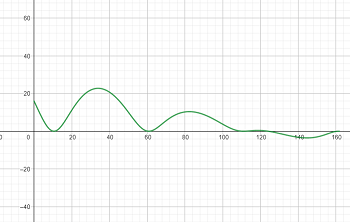# The path traveled by a car on the amusement park ride, the Wildcat, over the time interval 0...

## Question:

The path traveled by a car on the amusement park ride, the Wildcat, over the time interval {eq}0 \leq t \leq 20.5 {/eq} is modeled by the position vector {eq}\mathbf h(t) = \langle 8t + 2\cos t - 2, (16-t)(1-\sin t) \rangle {/eq}, where {eq}h(t) {/eq} his measured in yards.

a. Find the slope of the path at time {eq}t=5 {/eq}.

b. Find the speeds in yards per second when the car is at its maximum height and when the car is at its minimum height.

## Parametric Equations:

Recall that the slope is given by the value of the derivative at a point. To compute the slope given a set of parametric equation, we take advantage of the chain rule:

{eq}\begin{align*} \frac{dy}{dx} ****= \frac{dy/dt}{dx/dt} \end{align*} {/eq}

Note that for equations like the one above, the {eq}x {/eq} coordinate describes horizontal distance, and {eq}y {/eq} describes vertical distance, i.e. height.

Here's the roller coaster path:Part A

The derivative is

{eq}\begin{align*} \frac{dy}{dx} &= \frac{dy/dt}{dx/dt} \\ &= \frac{ \frac{d}{dt} \left( (16-t)(1-\sin t) \right)}{\frac{d}{dt} \left( 8t + 2\cos t - 2 \right)} \\ &= \frac{(-1)(1-\sin t) + (16-t)(-\cos t)}{8 - 2\sin t} \\ &= \frac{\sin t - 1 - (16-t) \cos t}{8 - 2\sin t} \end{align*} {/eq}

So when {eq}t = 5 {/eq}, the slope is

{eq}\begin{align*} y'(5) &= \frac{\sin 5 - 1 - (16-5) \cos 5}{8 - 2\sin 5} \\ &\approx -0.51 \end{align*} {/eq}

Part B

Since {eq}y {/eq} is the height, we want to find its extrema. We already found {eq}\frac{dy}{dt} = \sin t - 1 - (16-t) \cos t {/eq}, so extrema occur when

{eq}\begin{align*} \sin t - 1 - (16-t) \cos t &= 0 \end{align*} {/eq}

which we have no interest in solving analytically at all. Fortunately, we plotted the function, so we can use the trace function to see that the maximum height is at about {eq}t \approx 4.54 {/eq} and the minimum height is at about {eq}t \approx 18.15 {/eq}.

The velocity function is

{eq}\begin{align*} \vec v &= \left< \frac{dx}{dt} , \frac{dy}{dt}\right> \\ &= \left< 8 - 2\sin t,\ \sin t - 1 - (16-t) \cos t \right> \end{align*} {/eq}

Then the velocity at the max height is

{eq}\begin{align*} \vec v_{max} &= \left< 8 - 2\sin 4.54,\ \sin 4.54 - 1 - (16-4.54) \cos 4.54 \right> \\ &\approx \left< 9.97, 0 \right> \end{align*} {/eq}

which is a speed of about 10.0 yds/sec.

And the velocity at the min height is

{eq}\begin{align*} \vec v_{min} &= \left< 8 - 2\sin 18.15,\ \sin 18.15 - 1 - (16-18.15) \cos 18.15 \right> \\ &\approx \left< 9.29, 0 \right> \end{align*} {/eq}

which is a speed of about 9.3 yds/sec.